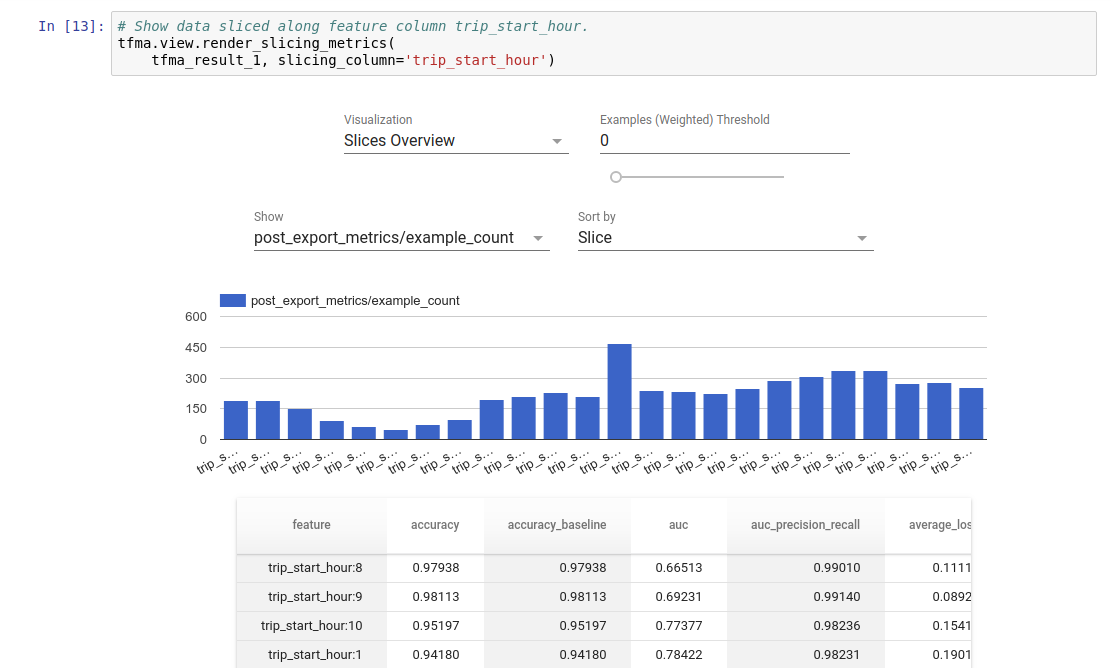# Getting Started with TensorFlow Model Analysis

• For: Machine Learning Engineers or Data Scientists
• who: want to analyze and understand their TensorFlow models
• it is: a standalone library or component of a TFx pipeline
• that: evaluates models on large amounts of data in a distributed manner on the same metrics defined in training. These metrics are compared over slices of data, and visualized in Jupyter or Colab notebooks.
• unlike: some model introspection tools like tensorboard that offer model introspection

TFMA performs its computations in a distributed manner over large amounts of data using Apache Beam. The following sections describe how to setup a basic TFMA evaluation pipeline. See architecture more details on the underlying implementation.

## Model Types Supported

TFMA is designed to support tensorflow based models, but can be easily extended to support other frameworks as well. Historically, TFMA required an `EvalSavedModel` be created to use TFMA, but the latest version of TFMA supports multiple types of models depending on the user's needs. Setting up an EvalSavedModel should only be required if a `tf.estimator` based model is used and custom training time metrics are required.

Note that because TFMA now runs based on the serving model, TFMA will no longer automatically evaluate metrics added at training time. The exception to this case is if a keras model is used since keras saves the metrics used along side of the saved model. However, if this is a hard requirement, the latest TFMA is backwards compatible such at an `EvalSavedModel` can still be run in a TFMA pipeline.

The following table summarizes the models supported by default:

Model Type Standard Training Metrics Custom Training Metrics Standard Post Training Metrics Custom Post Training Metrics
TF (keras) Y Not supported yet. Y Y
TF2 Signatures N/A N/A Y Y
EvalSavedModel (estimator) Y Y Y Y
• Standard metrics refers to metrics that are defined based only on label (i.e. `y_true`), prediction (i.e. `y_pred`), and example weight (i.e. `sample_weight`).
• Training metrics refers to metrics defined at training time and saved with the model (either TFMA EvalSavedModel or keras saved model).

See FAQ for more information no how to setup and configure these different model types.

## Example

The following uses `tfma.run_model_analysis` to perform evaluation on a serving model. For an explanation of the different settings needed see the setup guide. To run with an `EvalSavedModel`, just set `signature_name: "eval"` in the model spec.

Note this uses Beam's local runner which is mainly for local, small-scale experimentation.

``````# Run in a Jupyter Notebook.

eval_config = text_format.Parse("""
model_specs {
# This assumes a serving model with a "serving_default" signature.
label_key: "label"
example_weight_key: "weight"
}
metrics_spec {
# This assumes a binary classification model.
metrics { class_name: "AUC" }
... other metrics ...
}
slicing_specs {}
slicing_specs {
feature_keys: ["age"]
}
}
""", tfma.EvalConfig())

eval_shared_model = tfma.default_eval_shared_model(
eval_saved_model_path='/path/to/saved/model', tags=[tf.saved_model.SERVING])

eval_result = tfma.run_model_analysis(
eval_shared_model=eval_shared_model,
eval_config=eval_config,
# This assumes your data is a TFRecords file containing records in the
# tf.train.Example format.
data_location="/path/to/file/containing/tfrecords",
output_path="/path/for/metrics_for_slice_proto")

tfma.view.render_slicing_metrics(eval_result)
``````

For distributed evaluation, construct an Apache Beam pipeline using a distributed runner. In the pipeline, use the `tfma.ExtractEvaluateAndWriteResults` for evaluation and to write out the results. The results can be loaded for visualization using `tfma.load_eval_result`. For example:

``````# To run the pipeline.

eval_config = text_format.Parse("""
model_specs {
# This assumes a serving model with a "serving_default" signature.
label_key: "label"
example_weight_key: "weight"
}
metrics_specs {
# This assumes a binary classification model.
metrics { class_name: "AUC" }
... other metrics ...
}
slicing_specs {}
slicing_specs {
feature_keys: ["age"]
}
}
""", tfma.EvalConfig())

eval_shared_model = tfma.default_eval_shared_model(
eval_saved_model_path='/path/to/saved/model', eval_config=eval_config)

with beam.Pipeline(runner=...) as p:
_ = (p
# You can change the source as appropriate, e.g. read from BigQuery.
# This assumes your data is a TFRecords file containing records in the
# tf.train.Example format.
"/path/to/file/containing/tfrecords")
| 'ExtractEvaluateAndWriteResults' >>
tfma.ExtractEvaluateAndWriteResults(
eval_shared_model=eval_shared_model,
eval_config=eval_config,
output_path="/path/for/metrics_for_slice_proto"))

# To load and visualize results.
# Note that this code should be run in a Jupyter Notebook..